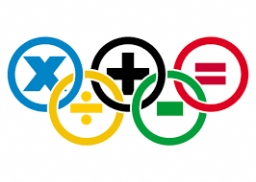# Probability 30421

There are 25 students in the class, 12 of whom are not ready for math. There are five students in the math class. What is the probability of at least 3 being math ready?

p =  0.4625

### Step-by-step explanation:Did you find an error or inaccuracy? Feel free to write us. Thank you!

Tips for related online calculators
Looking for a statistical calculator?
Would you like to compute the count of combinations?